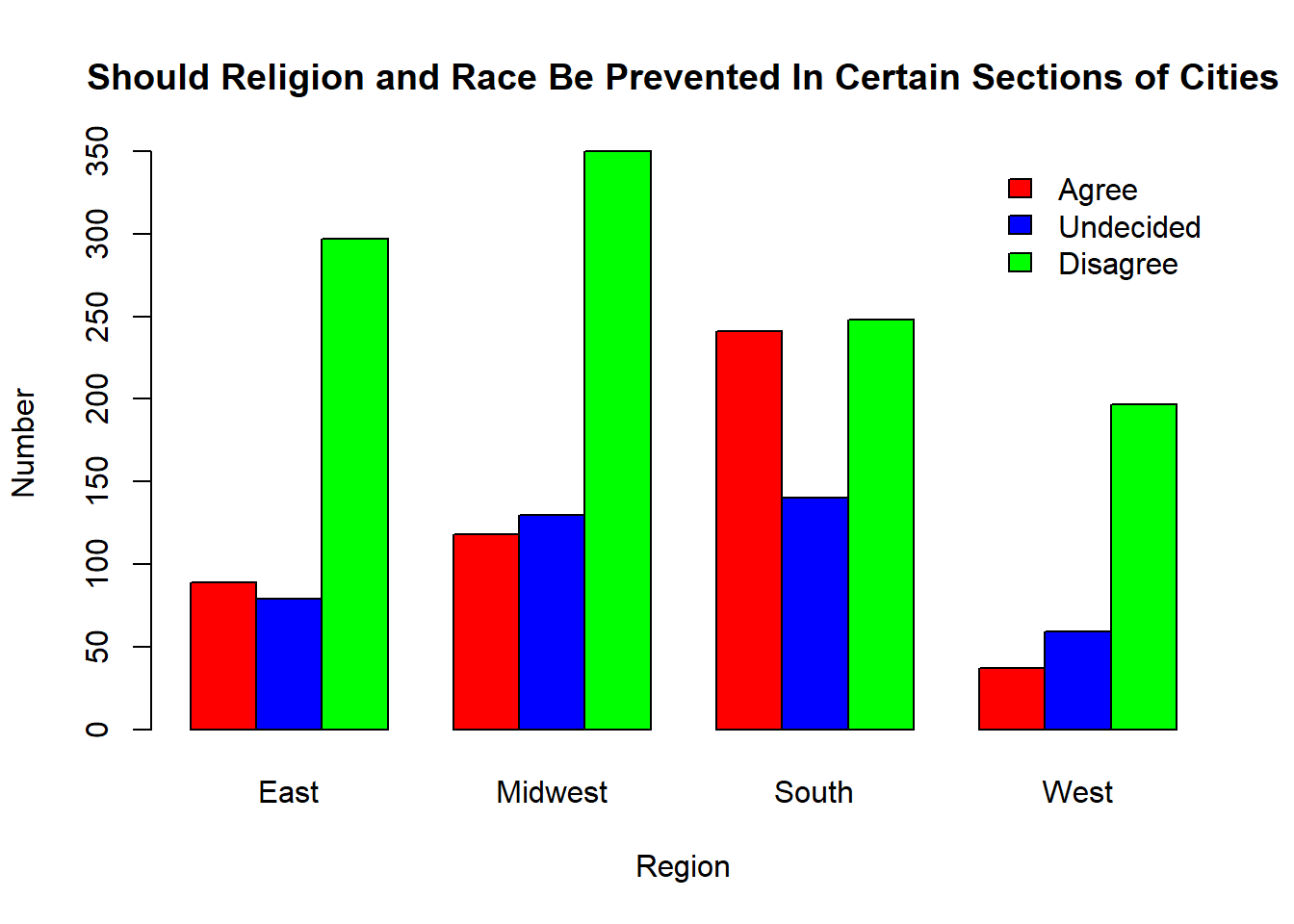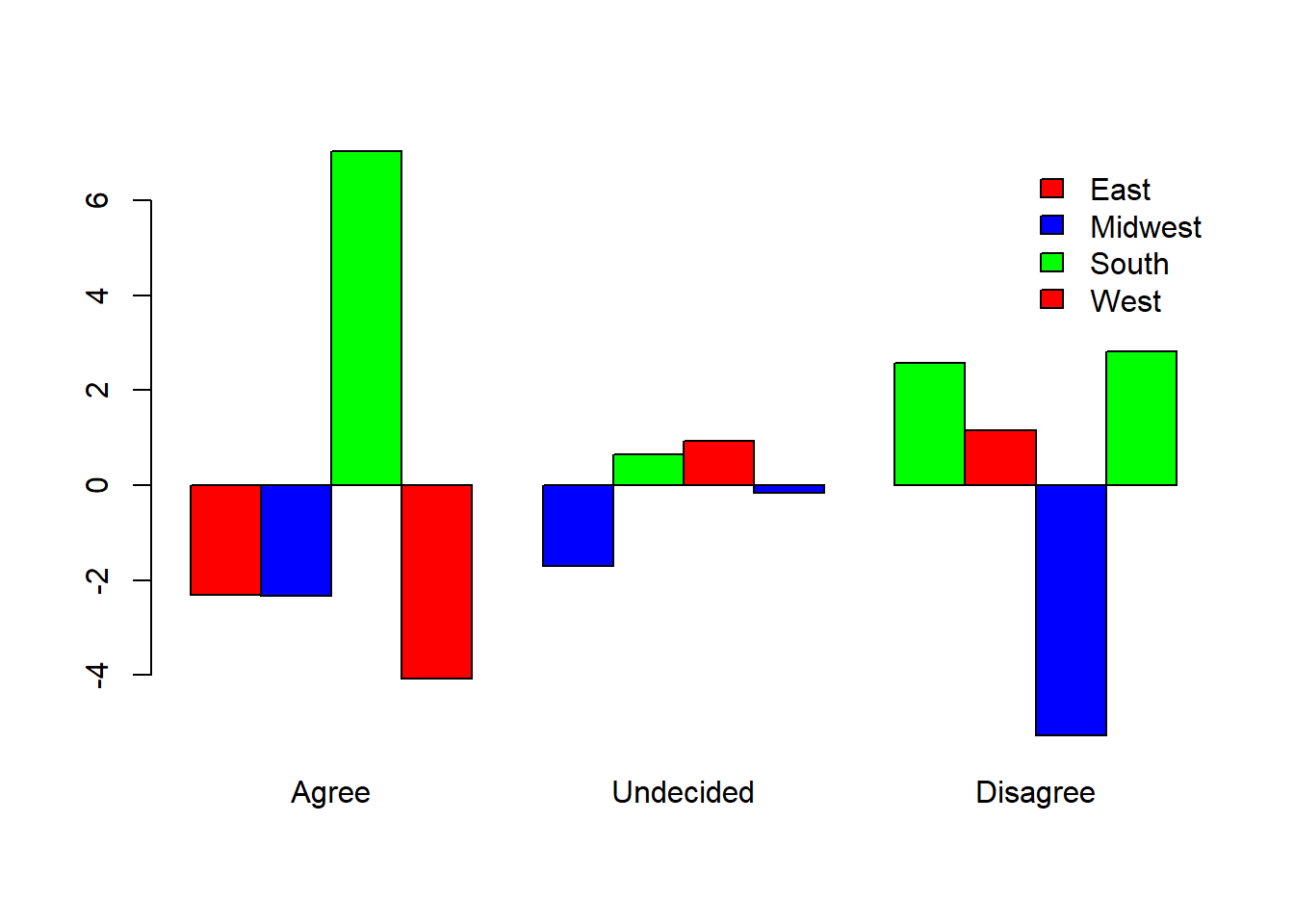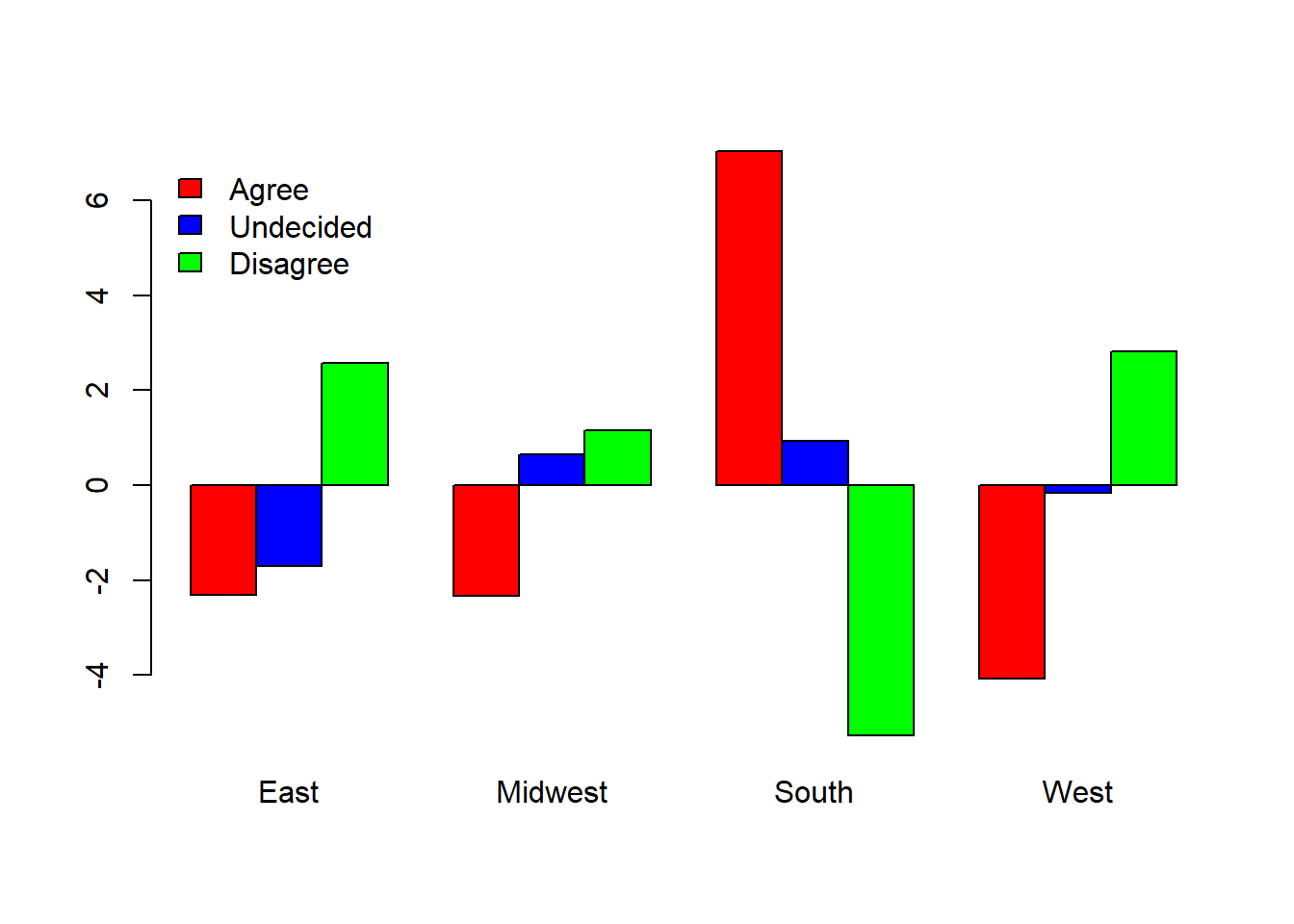### Background

Sometime around 1965 a survey was conducted where students at a certain university were asked the following question. “Do you think that some racial and religious groups should be prevented from living in certain sections of cities?” A summary of their response are recorded in the following table. The region of the United States that the student respondent was from was also recorded.

Region Agree Undecided Disagree
East 89 79 297
Midwest 118 130 350
South 241 140 248
West 37 59 197
discrim <- matrix(c(89,79,297,118,130,350,241,140,248,37,59,197), ncol = 3, byrow = T)
colnames(discrim) <- c("Agree","Undecided","Disagree")
rownames(discrim) <- c("East","Midwest","South","West")

### Questions & Hypotheses

For my chi-squared test, I will ask the question:

Is there an association between region and discrimination?

The hypotheses of this Chi-squared Test are written as:

$H_0: \text{Region and discrimination are independent.}$ $H_a: \text{Region and discrimination are associated (not independent).}$

The level of significance is:

$$α=0.05$$

### Analysis

I will use a Chi-squared Test to determine their association

chi.discrim <- chisq.test(discrim)
pander(chi.discrim)
Pearson’s Chi-squared test: discrim
Test statistic df P value
125 6 1.476e-24 * * *

The P-Value of the test appears to be $$1.476e-24$$, which gives us sufficient evidence to reject the null hypothesis.

Now it is time to see if this test is appropriate. All expected counts must be greater than 5. Then we can say that the requirements to run a Chi-Squared test are met.

pander(chi.discrim$expected > 5) Agree Undecided Disagree East TRUE TRUE TRUE Midwest TRUE TRUE TRUE South TRUE TRUE TRUE West TRUE TRUE TRUE Now I can create a barplot to help us visualize the data. We see that there is a relationship between region and opinion, also taking into account the p-value. From the plot, we are able to see that the East, Mid-West, and West have a similar pattern, as stated above. Although, we see that the South has about an even opinion of agreeing and disagreeing. barplot(t(discrim), beside=TRUE, legend.text=TRUE, args.legend = list(x="topright", bty = "n"),xlab="Region", ylab="Number",main="Should Religion and Race Be Prevented In Certain Sections of Cities", col=c("red","blue","green"))### Interpretation To interpret this test even further we need to look at the Pearson Residuals. We can see that the, from the South, we expected them to disagree with the opinion, but they were highly agreed. Taking into consideration the time and location when this study was done, it is to be expected with all of the segregation and unequal rights that were prevalent in the United States, and especially the South. pander(chi.discrim$residuals)
Agree Undecided Disagree
East -2.309 -1.696 2.575
Midwest -2.326 0.6392 1.159
South 7.043 0.9423 -5.27
West -4.088 -0.1577 2.821
barplot(chi.discrim$residuals, beside=TRUE, legend.text = TRUE, args.legend = list(x="topright", bty="n"), col=c("red","blue","green"))barplot(t(chi.discrim$residuals), beside=TRUE, legend.text = TRUE, args.legend = list(x="topleft", bty="n"), col=c("red","blue","green"))As we look at the two plots above, we can conclude that the South has about an even opinion of agreeing and disagreeing in regards to discrimination, while the undecided is close to what we were expecting. Looking at the West, we can conclude that they had the most drastic differences between the opinions. From the plots, we can see that the West has the least number of agreeing opinion, which can lead us to conclude that the West is most likely one of the most accepting and non-discriminatory regions in the United States. Overall, the West has the least amount that agrees to discrimination while the South had the highest amount of the agreeing opinion, which concludes that discrimination is most prevalent in the South.